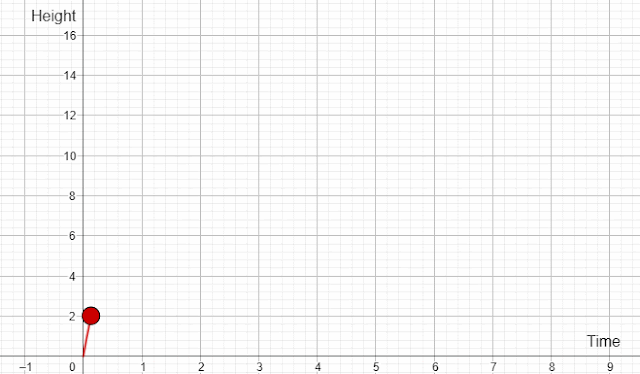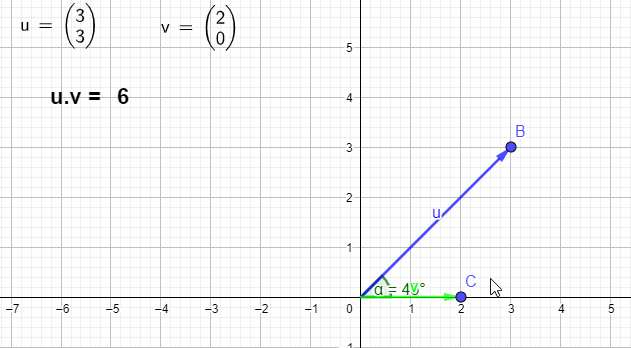## Posts

Showing posts from April 10, 2022

### Modelling with Differentiation for A Level Maths and PhysicsMotion under gravity - modelling with differentiation The motion of an object under gravity can easily be modelled by differentiation. Suppose the motion of a stone is given by the formula, y = 16t - 4t 2 , where y and t are displacement and time respectively.  y = 16t - 4t 2 dy/dt = 16 - 8t When the stone reaches the highest point, dy/dt = 0 16 - 8t = 0 8t = 16 t = 2s Maximum height reached by the stone = 16x2 - 4x2 2 = 16m d 2 y/dx 2 = -8 < 0 Therefore, when t = 2s, the height reaches the maximum. In order to play with the simulation interactively, please use the following and play with the slider:

### The dot product of two vectors: for A Level Further MathsThe dot product or scaler product of two vectors, a and b, is defined as follows: a.b = |a| |b| cos θ Since |a|, |b| and cos t are scalers, the dot product is a scaler. The dot product of unit vectors i.i = |i| |i| cos 0 = 1 X 1 X 1 = 1 i.j = |i| |j| cos 90 = 1 X 1 X 0 = 0 j.j = |j| |j| cos 0 = 1 X 1 X 1 = 1 The dot product of two perpendicular vectors is zero. E.g. a = 3i + 4j   b = 5i - 12j  θ = 60 |a| = 5   |b| = 13 a.b = |a| |b| cos θ a.b = 5 X13 X cos 60 a.b = 32.5 For more interactive simulations and worked examples, please use this link :